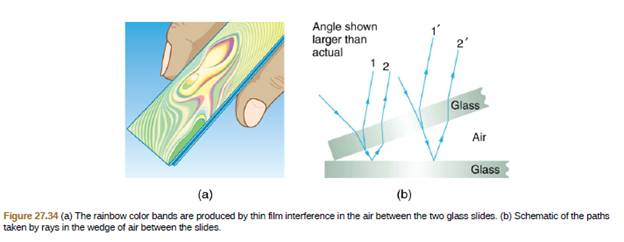Chapter 27, Problem 80PE

Chapter
Section
Textbook Problem

Figure 27.34 shows two 7.50-cm-long glass slides illuminated by pure 589-nm wavelength light incident perpendicularly. The top slide touches the bottom slide at one end and rests on some debris at the other end, forming a wedge of air. How thick is the debris, if the dark bands are 1.00 mm apart?To determine

Thickness of the debris

Explanation

Given info:

Length of the slide, l=7.50cm

Wavelength of the light, λ=589nm

Distance between the bands, d=1mm

Formula used:

The distance of the slide is calculated by the formula

dsinθ=λ

Here,

λ is the wavelength

d is the distance of the slide

θ is the angle between glass slide

Calculation:

The angle between the slide is calculated by the formula

dsinθ=λ

Plugging the values in the above equation

1×103sinθ=589×109θ=0

Still sussing out bartleby?

Check out a sample textbook solution.

See a sample solution

The Solution to Your Study Problems

Bartleby provides explanations to thousands of textbook problems written by our experts, many with advanced degrees!

Get Started